#### X-Ray Orders

This document looks at some data involving the X-Ray orders at a major hospital.

### STAT Orders

##   mean(Ordered.to.Complete...Mins)
## 1                         32.42555
##   sd(Ordered.to.Complete...Mins)
## 1                       24.91425

This set of data looks at all the STAT X-Ray orders from the data set RadDat.csv. The mean amount of time that a STAT priority X-ray took to be filled was 32.43 Minutes. The standard deviation of time taken to complete a STAT order was 24.91 Minutes. Displayed is a Histogram and Box Plot of the STAT orders.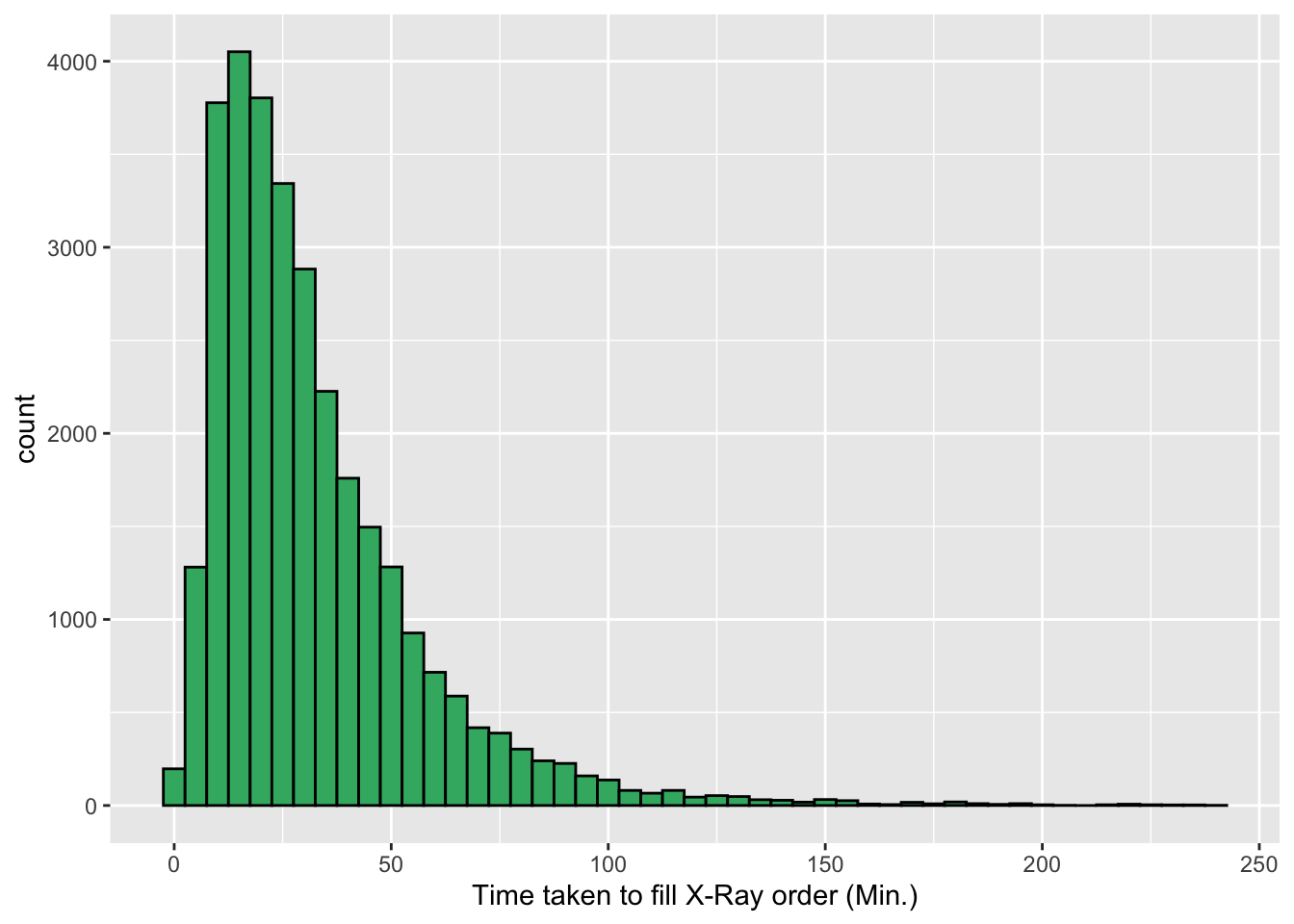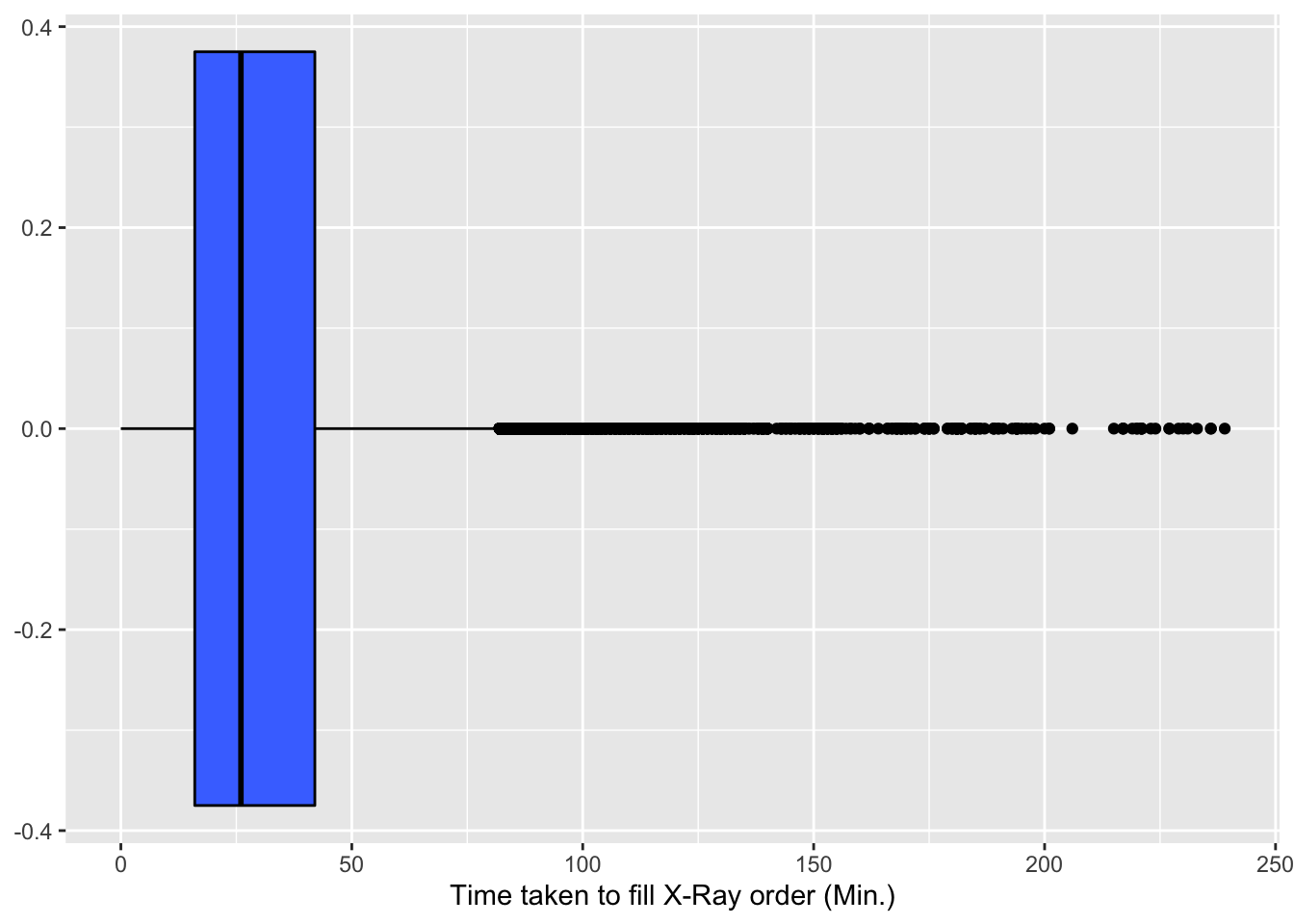### Routine Orders

##   mean(Ordered.to.Complete...Mins)
## 1                         128.4568
##   sd(Ordered.to.Complete...Mins)
## 1                       394.9069

This set of data shows the number of Routine orders completed by technician 65. The mean amount of time that it took technician 65 to fill orders was 128.46 Minutes. The standard deviation of his/her time to complete orders was 394.91 Minutes. The data in the following boxplot shows all of the orders that were requested, and how long it took them to be filled.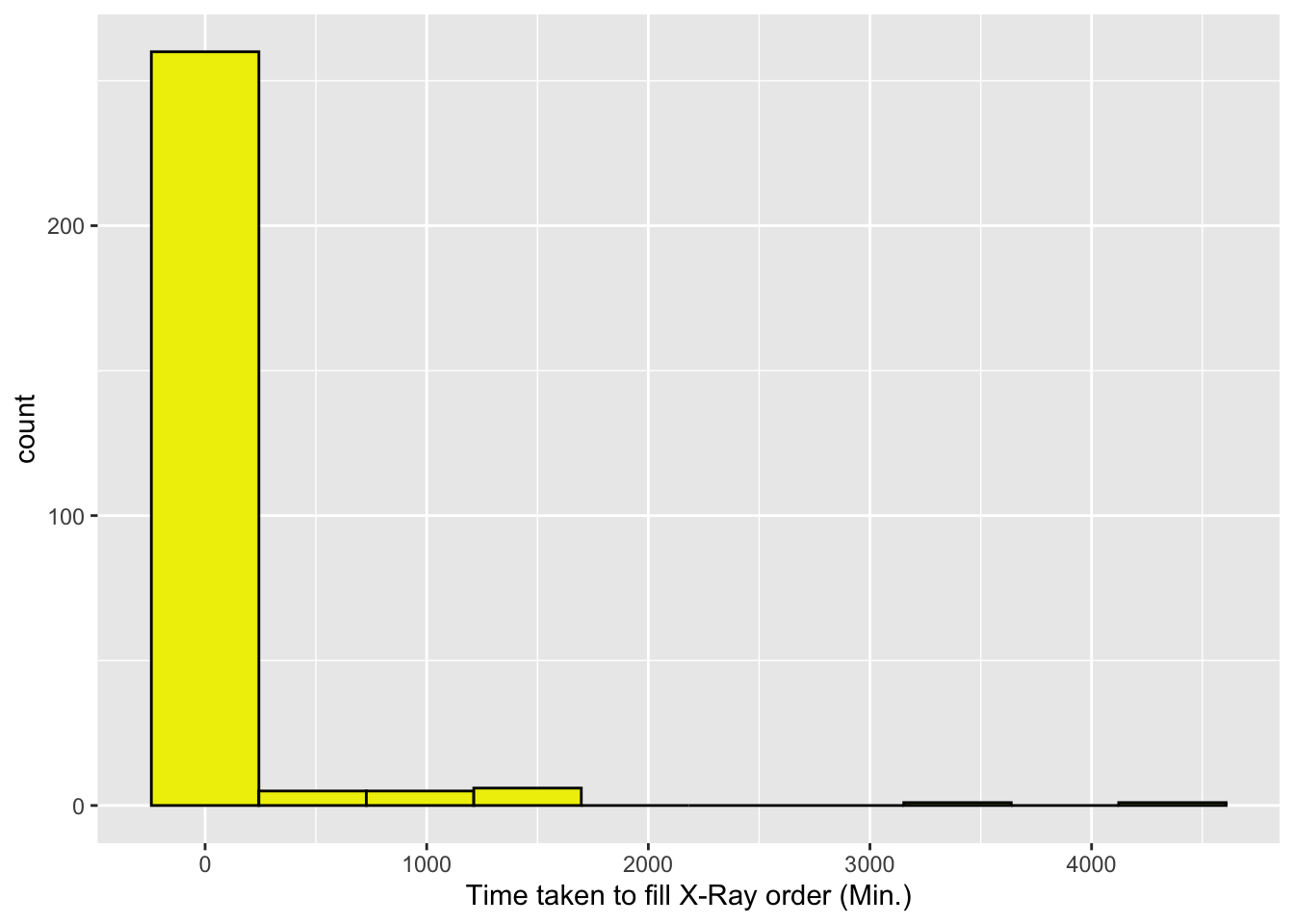### Location of Orders

The following column chart shows the time it took to complete orders at 6 different hospital wings.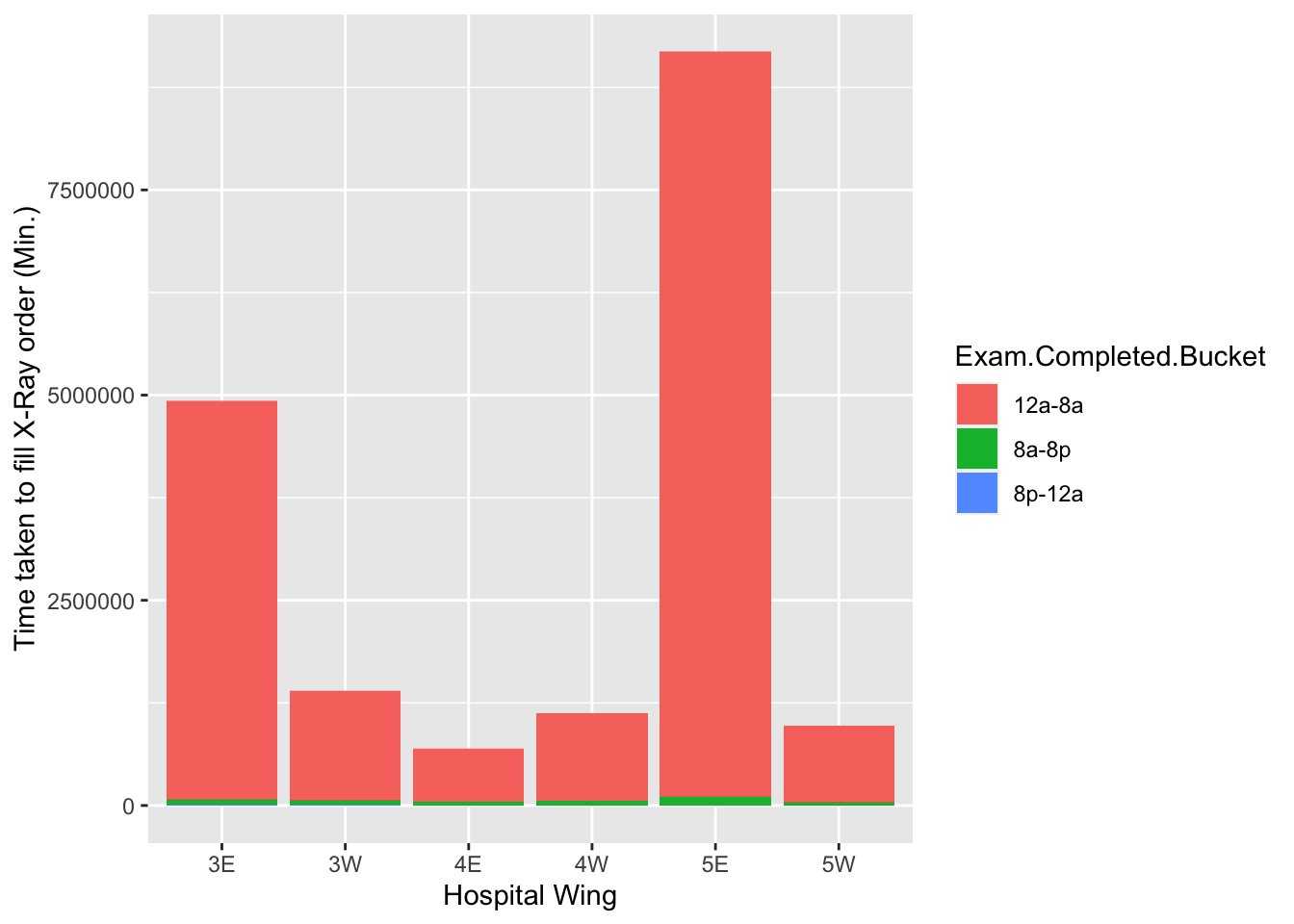### Orders filled within 4 hours of request

This histogram shows all of the orders that were filled in less than four hours, and it is faceted by the priority of the request.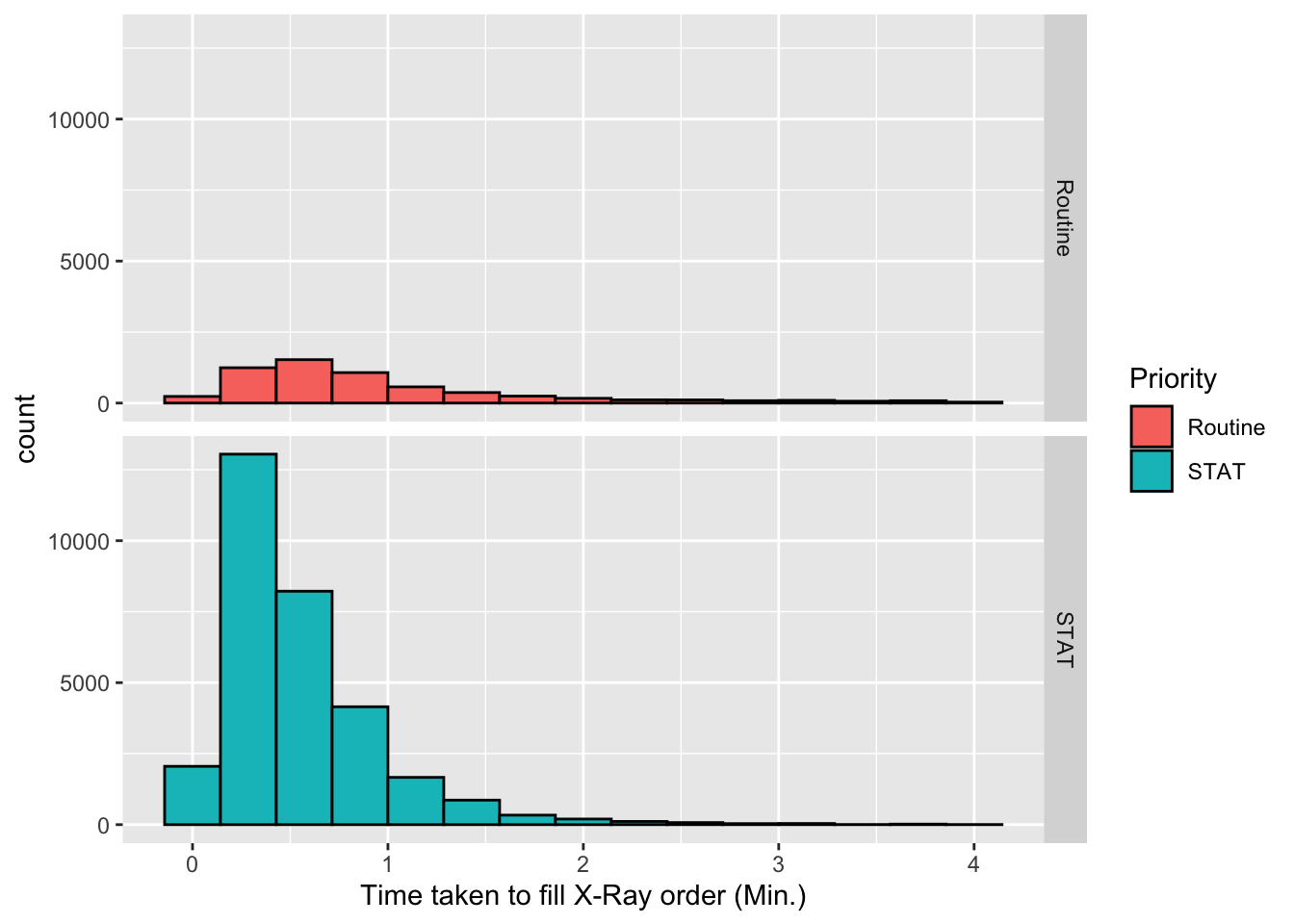knitr::opts_chunk\$set(echo = TRUE)
library(ggplot2)
library(dplyr)
STAT<-D %>% filter(Priority=="STAT") %>%
filter(Ordered.to.Complete...Hours<=4&Ordered.to.Complete...Hours>=0)
STAT2<-STAT %>% summarise(mean(Ordered.to.Complete...Mins))
STAT3<-STAT %>% summarise(sd(Ordered.to.Complete...Mins))
ggplot(data=STAT,aes(x=Ordered.to.Complete...Mins))+
geom_histogram(binwidth = 5,fill="mediumseagreen",color="black")+
xlab("Time taken to fill X-Ray order (Min.)")
ggplot(data=STAT,aes(x=Ordered.to.Complete...Mins))+
geom_boxplot(fill="royalblue1",color="black")+
xlab("Time taken to fill X-Ray order (Min.)")
Routine<-D %>% filter(Priority=="Routine") %>%
xlab("Time taken to fill X-Ray order (Min.)")